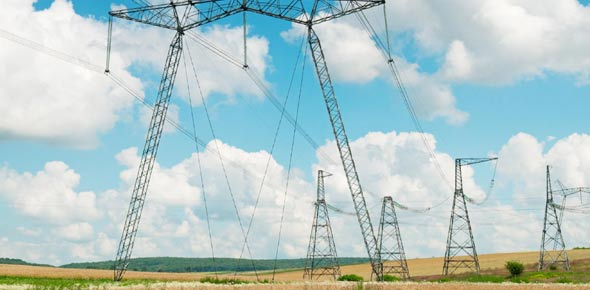# Electricity & Unit 1 Review

17 Questions | Total Attempts: 54SettingsLet's review Electricity! And Unit 1!

• 1.
Electricity is the flow of these:
• A.

Neutrons

• B.

Protons

• C.

Electrons

• D.

All of the above because they all make up the atoms

• 2.
In the static electricity lab, which of the following was true?
• A.

The friction between your hair and the balloon caused your hair to lose electrons and the negatively charged balloon was then attracted to your positively charged hair.

• B.

The friction between your hair and the balloon caused your hair to gain electrons and the negatively charged balloon was then attracted to your positively charged hair.

• C.

The friction between your hair and the balloon caused your hair to lose electrons and the positively charged balloon was then attracted to your positively charged hair.

• D.

The friction between your hair and the balloon caused your hair to lose electrons and the negatively charged balloon was then attracted to your negatively charged hair.

• 3.
The density of an object that takes up 10 mL of space and has a mass of 5 grams would be:
• A.

2 g/mL

• B.

0.5 g/mL

• C.

0.5 g*mL

• D.

2 g*mL

• 4.
True/False:  A Leyden jar could kill you.
• A.

True

• B.

False

• 5.
The roses smelled lovely is an example of:
• A.

Qualitative data

• B.

Quantitative data

• 6.
Lightning forms when:
• A.

The bottoms of clouds become positively charged and attract electrons from the surface of the earth.

• B.

Storms cause electrons to be ionized from air molecules and when they all happen in a straight line . . . uh oh!

• C.

Zeus causes it to happen.

• D.

There is a potential difference between the top and bottom of a cloud which causes streamers to form near the earth and lightning happens when the electrons are able to flow from the cloud to the earth.

• 7.
The resistance flowing through a 120 Volt circuit with 10 Amps of current would be:
• A.

1200 Ohms

• B.

12 Ohms

• C.

1200 Watts

• D.

12 Watts

• 8.
Electrical power is calculated by multiplying the:
• A.

Current X Resistance

• B.

Current X Voltage

• C.

Voltage X Resistance

• D.

Coulombs X Voltage

• 9.
The reason you could use a pickle light for a light bulb is because:
• A.

The arcing between the nails makes protons glow orange when electricity runs through the pickle.

• B.

You can use any sort of fruit or vegetable as a light bulb, they will all glow orange.

• C.

Electrons in the sodium in the pickle get excited and jump to higher energy levels releasing photons of light when they fall back down.

• 10.
The most common type of electricity in the US home is:
• A.

AC--120 Volts

• B.

DC--120 Volts

• C.

AC--240 Volts

• D.

DC--240 Volts

• 11.
A source of alternating current would be:
• A.

A wet cell

• B.

A dry cell

• C.

A wall socket

• D.

A flashlight

• 12.
What is the formula for density?:
• A.

Mass x volume

• B.

Mass/volume

• C.

Grams/centimeters

• D.

Volume/mass

• 13.
How much current flows through a flashlight if the battery has a resistance of 9 Ohms and runs off an 18 volt battery?
• A.

0.5 Amps

• B.

0.5 Ohms

• C.

2 Ohms

• D.

2 Amps

• 14.
True/False:  Theories explain WHAT happens, laws describe why things happen.
• A.

True

• B.

False

• 15.
A circuit is:
• A.

An open path through which protons flow.

• B.

An open path through which electrons flow.

• C.

A closed path through which protons flow.

• D.

A closed path through which electrons flow.

• 16.
A series circuit has:
• A.

One possible path for electricity to flow.

• B.

Two possible paths for electricity to flow.

• C.

Three or more possible paths for electricity to flow.

• 17.
A lightbulb lights because:
• A.

Electrons flow easily through the filament made of Tungsten.

• B.

Electrons have a hard time flowing through the filament.

• C.

Electrons rush through the filament and release photons of white light.

Related TopicsBack to top